# CBSEtips.in

### This is default featured slide 1 title

Go to Blogger edit html and find these sentences.Now replace these sentences with your own descriptions.This theme is Bloggerized by Lasantha Bandara - Premiumbloggertemplates.com.

### This is default featured slide 2 title

Go to Blogger edit html and find these sentences.Now replace these sentences with your own descriptions.This theme is Bloggerized by Lasantha Bandara - Premiumbloggertemplates.com.

### This is default featured slide 3 title

Go to Blogger edit html and find these sentences.Now replace these sentences with your own descriptions.This theme is Bloggerized by Lasantha Bandara - Premiumbloggertemplates.com.

### This is default featured slide 4 title

Go to Blogger edit html and find these sentences.Now replace these sentences with your own descriptions.This theme is Bloggerized by Lasantha Bandara - Premiumbloggertemplates.com.

### This is default featured slide 5 title

Go to Blogger edit html and find these sentences.Now replace these sentences with your own descriptions.This theme is Bloggerized by Lasantha Bandara - Premiumbloggertemplates.com.

## Sunday, 28 February 2021

### CBSE Class 6 Social - MCQ and Online Tests - History - Unit 2 - On The Trial of the Earliest People

#### CBSE Class 6 Social – MCQ and Online Tests – History – Unit 2 – On The Trial of the Earliest People

Every year CBSE students attend Annual Assessment exams for 6,7,8,9,11th standards. These exams are very competitive to all the students. So our website provides online tests for all the 6,7,8,9,11th standards’ subjects. These tests are also very effective and useful for those who preparing for any competitive exams like Olympiad etc. It can boost their preparation level and confidence level by attempting these chapter wise online tests.

These online tests are based on latest CBSE syllabus. While attempting these, our students can identify their weak lessons and continuously practice those lessons for attaining high marks. It also helps to revise the NCERT textbooks thoroughlyShare:

### CBSE Class 6 Social - MCQ and Online Tests - History - Unit 1 - What, Where, How and When?

#### CBSE Class 6 Social – MCQ and Online Tests – History – Unit 1 – What, Where, How and When?

Every year CBSE students attend Annual Assessment exams for 6,7,8,9,11th standards. These exams are very competitive to all the students. So our website provides online tests for all the 6,7,8,9,11th standards’ subjects. These tests are also very effective and useful for those who preparing for any competitive exams like Olympiad etc. It can boost their preparation level and confidence level by attempting these chapter wise online tests.

These online tests are based on latest CBSE syllabus. While attempting these, our students can identify their weak lessons and continuously practice those lessons for attaining high marks. It also helps to revise the NCERT textbooks thoroughlyShare:

### CBSE Class 6 Social - MCQ and Online Tests - Geography - Unit 8 - India Climate Vegetation and Wildlife

#### CBSE Class 6 Social – MCQ and Online Tests – Geography – Unit 8 – India Climate Vegetation and Wildlife

Every year CBSE students attend Annual Assessment exams for 6,7,8,9,11th standards. These exams are very competitive to all the students. So our website provides online tests for all the 6,7,8,9,11th standards’ subjects. These tests are also very effective and useful for those who preparing for any competitive exams like Olympiad etc. It can boost their preparation level and confidence level by attempting these chapter wise online tests.

These online tests are based on latest CBSE syllabus. While attempting these, our students can identify their weak lessons and continuously practice those lessons for attaining high marks. It also helps to revise the NCERT textbooks thoroughlyShare:

### CBSE Class 6 Social - MCQ and Online Tests - Geography - Unit 7 - Our Country India

#### CBSE Class 6 Social – MCQ and Online Tests – Geography – Unit 7 – Our Country India

Every year CBSE students attend Annual Assessment exams for 6,7,8,9,11th standards. These exams are very competitive to all the students. So our website provides online tests for all the 6,7,8,9,11th standards’ subjects. These tests are also very effective and useful for those who preparing for any competitive exams like Olympiad etc. It can boost their preparation level and confidence level by attempting these chapter wise online tests.

These online tests are based on latest CBSE syllabus. While attempting these, our students can identify their weak lessons and continuously practice those lessons for attaining high marks. It also helps to revise the NCERT textbooks thoroughlyShare:

### CBSE Class 6 Social - MCQ and Online Tests - Geography - Unit 6 - Major Landforms of the Earth

#### CBSE Class 6 Social – MCQ and Online Tests – Geography – Unit 6 – Major Landforms of the Earth

Every year CBSE students attend Annual Assessment exams for 6,7,8,9,11th standards. These exams are very competitive to all the students. So our website provides online tests for all the 6,7,8,9,11th standards’ subjects. These tests are also very effective and useful for those who preparing for any competitive exams like Olympiad etc. It can boost their preparation level and confidence level by attempting these chapter wise online tests.

These online tests are based on latest CBSE syllabus. While attempting these, our students can identify their weak lessons and continuously practice those lessons for attaining high marks. It also helps to revise the NCERT textbooks thoroughlyShare:

### CBSE Class 6 Social - MCQ and Online Tests - Geography - Unit 4 - Maps

#### CBSE Class 6 Social – MCQ and Online Tests – Geography – Unit 4 – Maps

Every year CBSE students attend Annual Assessment exams for 6,7,8,9,11th standards. These exams are very competitive to all the students. So our website provides online tests for all the 6,7,8,9,11th standards’ subjects. These tests are also very effective and useful for those who preparing for any competitive exams like Olympiad etc. It can boost their preparation level and confidence level by attempting these chapter wise online tests.

These online tests are based on latest CBSE syllabus. While attempting these, our students can identify their weak lessons and continuously practice those lessons for attaining high marks. It also helps to revise the NCERT textbooks thoroughlyShare:

### CBSE Class 6 Social - MCQ and Online Tests - Geography - Unit 5 - Major Domains of the Earth

#### CBSE Class 6 Social – MCQ and Online Tests – Geography – Unit 5 – Major Domains of the Earth

Every year CBSE students attend Annual Assessment exams for 6,7,8,9,11th standards. These exams are very competitive to all the students. So our website provides online tests for all the 6,7,8,9,11th standards’ subjects. These tests are also very effective and useful for those who preparing for any competitive exams like Olympiad etc. It can boost their preparation level and confidence level by attempting these chapter wise online tests.

These online tests are based on latest CBSE syllabus. While attempting these, our students can identify their weak lessons and continuously practice those lessons for attaining high marks. It also helps to revise the NCERT textbooks thoroughlyShare:

### CBSE Class 6 Social - MCQ and Online Tests - Geography - Unit 3 - Motions of the Earth

#### CBSE Class 6 Social – MCQ and Online Tests – Geography – Unit 3 – Motions of the Earth

Every year CBSE students attend Annual Assessment exams for 6,7,8,9,11th standards. These exams are very competitive to all the students. So our website provides online tests for all the 6,7,8,9,11th standards’ subjects. These tests are also very effective and useful for those who preparing for any competitive exams like Olympiad etc. It can boost their preparation level and confidence level by attempting these chapter wise online tests.

These online tests are based on latest CBSE syllabus. While attempting these, our students can identify their weak lessons and continuously practice those lessons for attaining high marks. It also helps to revise the NCERT textbooks thoroughlyShare:

### CBSE Class 6 Social - MCQ and Online Tests - Geography - Unit 2 - Globe Latitudes and Longitudes

#### CBSE Class 6 Social – MCQ and Online Tests – Geography – Unit 2 – Globe Latitudes and Longitudes

Every year CBSE students attend Annual Assessment exams for 6,7,8,9,11th standards. These exams are very competitive to all the students. So our website provides online tests for all the 6,7,8,9,11th standards’ subjects. These tests are also very effective and useful for those who preparing for any competitive exams like Olympiad etc. It can boost their preparation level and confidence level by attempting these chapter wise online tests.

These online tests are based on latest CBSE syllabus. While attempting these, our students can identify their weak lessons and continuously practice those lessons for attaining high marks. It also helps to revise the NCERT textbooks thoroughlyShare:

### CBSE Class 6 Social - MCQ and Online Tests - Geography - Unit 1 - The Earth in the Solar System

#### CBSE Class 6 Social – MCQ and Online Tests – Geography – Unit 1 – The Earth in the Solar System

Every year CBSE students attend Annual Assessment exams for 6,7,8,9,11th standards. These exams are very competitive to all the students. So our website provides online tests for all the 6,7,8,9,11th standards’ subjects. These tests are also very effective and useful for those who preparing for any competitive exams like Olympiad etc. It can boost their preparation level and confidence level by attempting these chapter wise online tests.

These online tests are based on latest CBSE syllabus. While attempting these, our students can identify their weak lessons and continuously practice those lessons for attaining high marks. It also helps to revise the NCERT textbooks thoroughlyShare:

### CBSE Class 6 Science - MCQ and Online Tests - Unit 16 - Garbage In Garbage Out

#### CBSE Class 6 Science – MCQ and Online Tests – Unit 16 – Garbage In Garbage Out

Every year CBSE students attend Annual Assessment exams for 6,7,8,9,11th standards. These exams are very competitive to all the students. So our website provides online tests for all the 6,7,8,9,11th standards’ subjects. These tests are also very effective and useful for those who preparing for any competitive exams like Olympiad etc. It can boost their preparation level and confidence level by attempting these chapter wise online tests.

These online tests are based on latest CBSE syllabus. While attempting these, our students can identify their weak lessons and continuously practice those lessons for attaining high marks. It also helps to revise the NCERT textbooks thoroughlyShare:

### CBSE Class 6 Science - MCQ and Online Tests - Unit 15 - Air Around Us

#### CBSE Class 6 Science – MCQ and Online Tests – Unit 15 – Air Around Us

Every year CBSE students attend Annual Assessment exams for 6,7,8,9,11th standards. These exams are very competitive to all the students. So our website provides online tests for all the 6,7,8,9,11th standards’ subjects. These tests are also very effective and useful for those who preparing for any competitive exams like Olympiad etc. It can boost their preparation level and confidence level by attempting these chapter wise online tests.

These online tests are based on latest CBSE syllabus. While attempting these, our students can identify their weak lessons and continuously practice those lessons for attaining high marks. It also helps to revise the NCERT textbooks thoroughlyShare:

### CBSE Class 6 Science - MCQ and Online Tests - Unit 14 - Water

#### CBSE Class 6 Science – MCQ and Online Tests – Unit 14 – Water

Every year CBSE students attend Annual Assessment exams for 6,7,8,9,11th standards. These exams are very competitive to all the students. So our website provides online tests for all the 6,7,8,9,11th standards’ subjects. These tests are also very effective and useful for those who preparing for any competitive exams like Olympiad etc. It can boost their preparation level and confidence level by attempting these chapter wise online tests.

These online tests are based on latest CBSE syllabus. While attempting these, our students can identify their weak lessons and continuously practice those lessons for attaining high marks. It also helps to revise the NCERT textbooks thoroughlyShare:

### CBSE Class 6 Science - MCQ and Online Tests - Unit 13 - Fun with Magnets

#### CBSE Class 6 Science – MCQ and Online Tests – Unit 13 – Fun with Magnets

Every year CBSE students attend Annual Assessment exams for 6,7,8,9,11th standards. These exams are very competitive to all the students. So our website provides online tests for all the 6,7,8,9,11th standards’ subjects. These tests are also very effective and useful for those who preparing for any competitive exams like Olympiad etc. It can boost their preparation level and confidence level by attempting these chapter wise online tests.

These online tests are based on latest CBSE syllabus. While attempting these, our students can identify their weak lessons and continuously practice those lessons for attaining high marks. It also helps to revise the NCERT textbooks thoroughlyShare:

### CBSE Class 6 Science - MCQ and Online Tests - Unit 11 - Light Shadows and Reflection

#### CBSE Class 6 Science – MCQ and Online Tests – Unit 11 – Light Shadows and Reflection

Every year CBSE students attend Annual Assessment exams for 6,7,8,9,11th standards. These exams are very competitive to all the students. So our website provides online tests for all the 6,7,8,9,11th standards’ subjects. These tests are also very effective and useful for those who preparing for any competitive exams like Olympiad etc. It can boost their preparation level and confidence level by attempting these chapter wise online tests.

These online tests are based on latest CBSE syllabus. While attempting these, our students can identify their weak lessons and continuously practice those lessons for attaining high marks. It also helps to revise the NCERT textbooks thoroughlyShare:

### CBSE Class 6 Science - MCQ and Online Tests - Unit 12 - Electricity and Circuits

#### CBSE Class 6 Science – MCQ and Online Tests – Unit 12 – Electricity and Circuits

Every year CBSE students attend Annual Assessment exams for 6,7,8,9,11th standards. These exams are very competitive to all the students. So our website provides online tests for all the 6,7,8,9,11th standards’ subjects. These tests are also very effective and useful for those who preparing for any competitive exams like Olympiad etc. It can boost their preparation level and confidence level by attempting these chapter wise online tests.

These online tests are based on latest CBSE syllabus. While attempting these, our students can identify their weak lessons and continuously practice those lessons for attaining high marks. It also helps to revise the NCERT textbooks thoroughlyShare:

### CBSE Class 6 Science - MCQ and Online Tests - Unit 8 - Body Movements

#### CBSE Class 6 Science – MCQ and Online Tests – Unit 8 – Body Movements

Every year CBSE students attend Annual Assessment exams for 6,7,8,9,11th standards. These exams are very competitive to all the students. So our website provides online tests for all the 6,7,8,9,11th standards’ subjects. These tests are also very effective and useful for those who preparing for any competitive exams like Olympiad etc. It can boost their preparation level and confidence level by attempting these chapter wise online tests.

These online tests are based on latest CBSE syllabus. While attempting these, our students can identify their weak lessons and continuously practice those lessons for attaining high marks. It also helps to revise the NCERT textbooks thoroughlyShare:

### CBSE Class 6 Science - MCQ and Online Tests - Unit 9 - The Living Organisms and Their Surroundings

#### CBSE Class 6 Science – MCQ and Online Tests – Unit 9 – The Living Organisms and Their Surroundings

Every year CBSE students attend Annual Assessment exams for 6,7,8,9,11th standards. These exams are very competitive to all the students. So our website provides online tests for all the 6,7,8,9,11th standards’ subjects. These tests are also very effective and useful for those who preparing for any competitive exams like Olympiad etc. It can boost their preparation level and confidence level by attempting these chapter wise online tests.

These online tests are based on latest CBSE syllabus. While attempting these, our students can identify their weak lessons and continuously practice those lessons for attaining high marks. It also helps to revise the NCERT textbooks thoroughlyShare:

### CBSE Class 6 Science - MCQ and Online Tests - Unit 10 - Motion and Measurement of Distances

#### CBSE Class 6 Science – MCQ and Online Tests – Unit 10 – Motion and Measurement of Distances

Every year CBSE students attend Annual Assessment exams for 6,7,8,9,11th standards. These exams are very competitive to all the students. So our website provides online tests for all the 6,7,8,9,11th standards’ subjects. These tests are also very effective and useful for those who preparing for any competitive exams like Olympiad etc. It can boost their preparation level and confidence level by attempting these chapter wise online tests.

These online tests are based on latest CBSE syllabus. While attempting these, our students can identify their weak lessons and continuously practice those lessons for attaining high marks. It also helps to revise the NCERT textbooks thoroughlyShare:

### CBSE Class 6 Science - MCQ and Online Tests - Unit 7 - Getting to Know Plants

#### CBSE Class 6 Science – MCQ and Online Tests – Unit 7 – Getting to Know Plants

Every year CBSE students attend Annual Assessment exams for 6,7,8,9,11th standards. These exams are very competitive to all the students. So our website provides online tests for all the 6,7,8,9,11th standards’ subjects. These tests are also very effective and useful for those who preparing for any competitive exams like Olympiad etc. It can boost their preparation level and confidence level by attempting these chapter wise online tests.

These online tests are based on latest CBSE syllabus. While attempting these, our students can identify their weak lessons and continuously practice those lessons for attaining high marks. It also helps to revise the NCERT textbooks thoroughlyShare:

### CBSE Class 6 Science - MCQ and Online Tests - Unit 6 - Changes Around Us

#### CBSE Class 6 Science – MCQ and Online Tests – Unit 6 – Changes Around Us

Every year CBSE students attend Annual Assessment exams for 6,7,8,9,11th standards. These exams are very competitive to all the students. So our website provides online tests for all the 6,7,8,9,11th standards’ subjects. These tests are also very effective and useful for those who preparing for any competitive exams like Olympiad etc. It can boost their preparation level and confidence level by attempting these chapter wise online tests.

These online tests are based on latest CBSE syllabus. While attempting these, our students can identify their weak lessons and continuously practice those lessons for attaining high marks. It also helps to revise the NCERT textbooks thoroughlyShare:

### CBSE Class 6 Science - MCQ and Online Tests - Unit 4 - Sorting Materials Into Groups

#### CBSE Class 6 Science – MCQ and Online Tests – Unit 4 – Sorting Materials Into Groups

Every year CBSE students attend Annual Assessment exams for 6,7,8,9,11th standards. These exams are very competitive to all the students. So our website provides online tests for all the 6,7,8,9,11th standards’ subjects. These tests are also very effective and useful for those who preparing for any competitive exams like Olympiad etc. It can boost their preparation level and confidence level by attempting these chapter wise online tests.

These online tests are based on latest CBSE syllabus. While attempting these, our students can identify their weak lessons and continuously practice those lessons for attaining high marks. It also helps to revise the NCERT textbooks thoroughly.Share:

### CBSE Class 6 Science - MCQ and Online Tests - Unit 5 - Separation of Substances

#### CBSE Class 6 Science – MCQ and Online Tests – Unit 5 – Separation of Substances

Every year CBSE students attend Annual Assessment exams for 6,7,8,9,11th standards. These exams are very competitive to all the students. So our website provides online tests for all the 6,7,8,9,11th standards’ subjects. These tests are also very effective and useful for those who preparing for any competitive exams like Olympiad etc. It can boost their preparation level and confidence level by attempting these chapter wise online tests.

These online tests are based on latest CBSE syllabus. While attempting these, our students can identify their weak lessons and continuously practice those lessons for attaining high marks. It also helps to revise the NCERT textbooks thoroughlyShare:

### CBSE Class 6 Science - MCQ and Online Tests - Unit 3 - Fibre to Fabric

#### CBSE Class 6 Science – MCQ and Online Tests – Unit 3 – Fibre to Fabric

Every year CBSE students attend Annual Assessment exams for 6,7,8,9,11th standards. These exams are very competitive to all the students. So our website provides online tests for all the 6,7,8,9,11th standards’ subjects. These tests are also very effective and useful for those who preparing for any competitive exams like Olympiad etc. It can boost their preparation level and confidence level by attempting these chapter wise online tests.

These online tests are based on latest CBSE syllabus. While attempting these, our students can identify their weak lessons and continuously practice those lessons for attaining high marks. It also helps to revise the NCERT textbooks thoroughly.Share:

### CBSE Class 6 Science - MCQ and Online Tests - Unit 2 - Components of Food

#### CBSE Class 6 Science – MCQ and Online Tests – Unit 2 – Components of Food

Every year CBSE students attend Annual Assessment exams for 6,7,8,9,11th standards. These exams are very competitive to all the students. So our website provides online tests for all the 6,7,8,9,11th standards’ subjects. These tests are also very effective and useful for those who preparing for any competitive exams like Olympiad etc. It can boost their preparation level and confidence level by attempting these chapter wise online tests.

These online tests are based on latest CBSE syllabus. While attempting these, our students can identify their weak lessons and continuously practice those lessons for attaining high marks. It also helps to revise the NCERT textbooks thoroughly.Share:

### CBSE Class 6 Science - MCQ and Online Tests - Unit 1 - Food Where Does It Come From

#### CBSE Class 6 Science – MCQ and Online Tests – Unit 1 – Food Where Does It Come From

Every year CBSE students attend Annual Assessment exams for 6,7,8,9,11th standards. These exams are very competitive to all the students. So our website provides online tests for all the 6,7,8,9,11th standards’ subjects. These tests are also very effective and useful for those who preparing for any competitive exams like Olympiad etc. It can boost their preparation level and confidence level by attempting these chapter wise online tests.

These online tests are based on latest CBSE syllabus. While attempting these, our students can identify their weak lessons and continuously practice those lessons for attaining high marks. It also helps to revise the NCERT textbooks thoroughly.Share:

### CBSE Class 6 Maths - MCQ and Online Tests - Unit 13 - Symmetry

#### CBSE Class 6 Maths – MCQ and Online Tests – Unit 13 – Symmetry

Every year CBSE students attend Annual Assessment exams for 6,7,8,9,11th standards. These exams are very competitive to all the students. So our website provides online tests for all the 6,7,8,9,11th standards’ subjects. These tests are also very effective and useful for those who preparing for any competitive exams like Olympiad etc. It can boost their preparation level and confidence level by attempting these chapter wise online tests.

These online tests are based on latest CBSE syllabus. While attempting these, our students can identify their weak lessons and continuously practice those lessons for attaining high marks. It also helps to revise the NCERT textbooks thoroughly.#### CBSE Class 6 Maths – MCQ and Online Tests – Unit 13 – Symmetry

Question 1.
Which of the following letters has horizontal line of symmetry?
(a) C
(b) A
(c) J
(d) L.

Question 2.
How many lines of symmetry does the figure have ?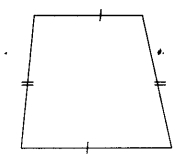(a) 1
(b) 2
(c) 3
(d) no line of symmetry

Question 3.
Which of the following letters has horizontal line of symmetry?
(a) Z
(b) V
(c) U
(d) E.

Question 4.
How many lines of symmetry does the figure have?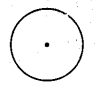(a) 1
(b) 2
(c) 3
(d) Countless.

Question 5.
Which of the following letters has vertical line of symmetry?
(a) R
(b) C
(c) B
(d) T.

Question 6.
How many lines of symmetry does the figure have?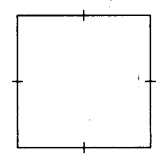(a) 1
(b) 2
(c) 3
(d) 4

Question 7.
How many lines of symmetry does the figure have ?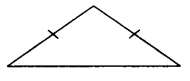(a) 1
(b) 2
(c) 3
(d) 4

Question 8.
How many lines of symmetry does the figure have?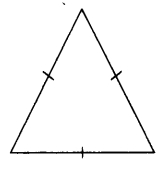(a) 1
(b) 2
(c) 3
(d) 4

Question 9.
How many lines of symmetry does the figure have?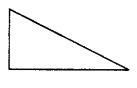(a) 0
(b) 1
(c) 2
(d) countless

Question 10.
How many lines of symmetry does the figure have?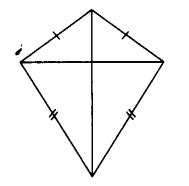(a) 1
(b) 2
(c) 3
(d) 4

Question 11.
How many lines of symmetry does the figure have?(a) 1
(b) 2
(c) 3
(d) 4

Question 12.
How many lines of symmetry does the figure have?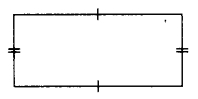(a) 1
(b) 2
(c) 3
(d) 4.

Question 13.
How many lines of symmetry does a regular hexagon have?
(a) 1
(b) 3
(c) 4
(d) 6

Question 14.
Which of the following letters has horizontal line of symmetry?
(a) S
(b) W
(c) D
(d) Y.

Question 15.
Which of the following letters has vertical line of symmetry?
(a) N
(b) K
(c) B
(d) M.

Share:

### CBSE Class 6 Maths - MCQ and Online Tests - Unit 14 - Practical Geometry

#### CBSE Class 6 Maths – MCQ and Online Tests – Unit 14 – Practical Geometry

Every year CBSE students attend Annual Assessment exams for 6,7,8,9,11th standards. These exams are very competitive to all the students. So our website provides online tests for all the 6,7,8,9,11th standards’ subjects. These tests are also very effective and useful for those who preparing for any competitive exams like Olympiad etc. It can boost their preparation level and confidence level by attempting these chapter wise online tests.

These online tests are based on latest CBSE syllabus. While attempting these, our students can identify their weak lessons and continuously practice those lessons for attaining high marks. It also helps to revise the NCERT textbooks thoroughly.#### CBSE Class 6 Maths – MCQ and Online Tests – Unit 14 – Practical Geometry

Question 1.
If two lines have only one point in common, what are they called?
(a) Parallel lines
(b) Intersecting lines
(c) Perpendicular lines
(d) Transversal

Hint:
Intersecting lines have only one point in common.

Question 2.
$$\overrightarrow { MN }$$ is the perpendicular bisector of $$\overleftrightarrow { AB }$$. Which of the given statements is correct?
(i) ∠ANM + ∠MNB = 90∘
(ii) $$\overline { AN }$$ = $$\overline { NB }$$
(iii) $$\overline { AN }$$ = 2 $$\overline { NB }$$
(iv) ∠MNB = $$\frac{1}{2}$$ ∠ANM
(a) (i) and (iii) only
(b) (ii) and (iv) only
(c) (i) and (ii) only
(d) (ii) and (iii) only

Answer: (c) (i) and (ii) only
Hint:
$$\overrightarrow { NM }$$ ⊥ $$\overleftrightarrow { AB }$$ and $$\overrightarrow { NM }$$ divides $$\overleftrightarrow { AB }$$ into two congruent parts.Clearly ∠ANM = ∠MNB = 90∘ is true. $$\overline { AN }$$ = $$\overline { NB }$$ is true since $$\overrightarrow { NM }$$ ⊥ $$\overleftrightarrow { AB }$$, $$\overline { AN }$$ = 2$$\overline { NB }$$ is false, and ∠MNB = $$\frac{1}{2}$$ ∠ANM is false. Thus, only (i) and (ii) are correct.

Question 3.
Which of the following can be drawn on a piece of paper?
(a) A line
(b) A line segment
(c) A ray
(d) A plane

Question 4.
Identify the uses of a ruler.
(a) To draw a line segment of a given length
(b) To draw a copy of a given segment.
(c) To draw a diameter of a circle.
(d) All the above.

Hint:
A ruler is used to draw a line segment of a given length, to draw the copy of a given segment, and to draw a diameter of a circle. Thus, all the given options are correct.

Question 5.
P is the midpoint of $$\overline { AB }$$. M and N are midpoints of $$\overline { AP }$$ respectively. What is the measure of $$\overline { MN }$$?
(a) $$\frac{1}{3}$$ $$\overline { AB }$$
(b) $$\frac{1}{2}$$ $$\overline { AB }$$
(c) $$\frac{1}{2}$$ $$\overline { AP }$$
(d) $$\frac{3}{2}$$ $$\overline { AB }$$

Answer: (b) $$\frac{1}{2}$$ $$\overline { AB }$$
Hint:P is the midpoint of $$\overline { AB }$$ ⇒ AP = PB M and N are midpoints of $$\overline { AP }$$ and $$\overline { PB }$$ ⇒ $$\overline { AM }$$ = $$\overline { MP }$$ and $$\overline { PN }$$ = $$\overline { NB }$$
∴ $$\overline { MN }$$ = $$\overline { MP }$$ + $$\overline { PN }$$ = $$\frac{1}{2}$$ $$\overline { AP }$$ + $$\frac{1}{2}$$ $$\overline { PB }$$ = $$\frac{1}{2}$$ ($$\overline { AP }$$ + $$\overline { PB }$$ ) = $$\frac{1}{2}$$ ($$\overline { AB }$$)

Question 6.
$$\overrightarrow { XY }$$ bisects∠AXB. If ∠YXB = 37.5∘, what is the measure of ∠AXB?
(a) 37.5°
(b) 74°
(c) 64°
(d) 75°

Question 7.
$$\overrightarrow { XY }$$ divides ∠MXN = 72° in the ratio 1 : 2. What is the measure of ∠YXN?(a) 48°
(b) 24°
(c) 72°
(d) 96°

Hint: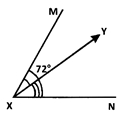Given ∠MXN = 72∘ and $$\overrightarrow { XY }$$ divides ∠MXN in the ratio 1 : 2.
∠YXN = $$\frac{2}{3}$$ ∠MXN = $$\frac{2}{3}$$ × 72° = 48°

Question 8.
X and Y are two distinct points in a plane. How many lines can be drawn passing through both X and Y?
(a) 0
(b) 1
(c) Only 2
(d) Infinitely many

Question 9.
l || m. P and Q are points on land m respectively such that PQ ⊥ lR is a point on a line n in the same plane such that $$\overline { PQ }$$ = $$\overline { QR }$$. Which of the following is true?
(a) l || n
(b) m || n
(c) Both [a] and [b]
(d) Neither [a] nor [b]

Answer: (c) Both [a] and [b]
Hint: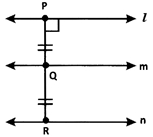Clearly, from the given data and the figure, l || n and m || n.

Question 10.
Lines a, b, p, q, m, n and x have a point P common to all of them. What is the name of P?
(a) Point of concurrence
(b) Point of intersection
(c) Common point
(d) Distinct point

Hint:
A point common to multiple lines is called a point of concurrence as the lines are concurrent lines.

Question 11.
$$\overrightarrow { QZ }$$ is the bisector of ∠PQZ = ∠PQR. Which of the following is true?
(a) ∠PQZ = ∠PQR
(b) ∠PQZ = ∠ZQR
(c) ∠PQZ = $$\frac{1}{2}$$ ∠ZQR
(d) Both [b] and [c]

Answer: (d) Both [b] and [c]
Hint:
$$\overrightarrow { QZ }$$ bisects ∠PQR (Given)Thus. ∠PQZ = ∠ZQR = $$\frac{1}{2}$$ ∠PQR

Question 12.
At 7 a.m. the angle between the Sun’s ray and the ground at a point is 43°. What would be the angle at 10 a.m.?
(a) 40°
(b) 90°
(c) Between 43° and 90°
(d) Greater than 90°

Answer: (c) Between 43° and 90°
Hint:Let QP be the sun’s ray and RP be the ground. The angle between QP and PR at P is 43° at 7 a.m. At 10 a.m., the sun’s ray is Q’P. We know that at 12 noon the sun is exactly above our head. So, the sun’s ray will be perpendicular to the ground. So, clearly at 10 am, the required angle will be between 43° and 90°.

Question 13.
Identify the pair of parallel lines.
(i) Lines m and n have two points in common.
(ii) Lines p and q do not have any point in common
(iii) Lines p and q have a point X in common.
(a) (i) and (ii) only
(b) (ii) only
(c) (ii) and (iii) only
(d) (i), (ii) and (iii)

Hint:
Parallel lines do not have any point in common.

Question 14.
A line segment $$\overrightarrow { TP }$$ is bisected at I. What is the measure of $$\overrightarrow { TI }$$?
(a) $$\frac{1}{2}$$$$\overrightarrow { TP }$$
(b) $$\overrightarrow { IP }$$
(c) $$\overrightarrow { TP }$$
(d) $$\frac{1}{3}$$ $$\overrightarrow { TP }$$

Answer: (b) $$\overrightarrow { IP }$$
Hint:
$$\overrightarrow { TI }$$ = $$\frac{1}{3}$$ $$\overrightarrow { TP }$$ = IP

Question 15.
Identify the one with no definite length.
(a) $$\overleftrightarrow { AB }$$
(b) $$\overline { PQ }$$
(c) -XYZ
(d) $$\overline { MN }$$

Answer: (a) $$\overleftrightarrow { AB }$$
Hint:
$$\overleftrightarrow { AB }$$ has no definite length.

Share:

### CBSE Class 6 Maths - MCQ and Online Tests - Unit 12 - Ratio and Proportion

#### CBSE Class 6 Maths – MCQ and Online Tests – Unit 12 – Ratio and Proportion

Every year CBSE students attend Annual Assessment exams for 6,7,8,9,11th standards. These exams are very competitive to all the students. So our website provides online tests for all the 6,7,8,9,11th standards’ subjects. These tests are also very effective and useful for those who preparing for any competitive exams like Olympiad etc. It can boost their preparation level and confidence level by attempting these chapter wise online tests.

These online tests are based on latest CBSE syllabus. While attempting these, our students can identify their weak lessons and continuously practice those lessons for attaining high marks. It also helps to revise the NCERT textbooks thoroughly.#### CBSE Class 6 Maths – MCQ and Online Tests – Unit 12 – Ratio and Proportion

Question 1.
The speed of Shubham is 6 km per hour. The speed of Yash is 2 km per hour. The ratio of the speed of Shubham to the speed of Yash is
(a) 2:3
(b) 3:1
(c) 1:3
(d) 3:2.

Hint:
$$\frac { 6 }{ 2 }$$ = $$\frac { 3 }{ 1 }$$ = 3 : 1

Question 2.
Which of the following are in proportion?
(a) 2,3,20,30
(b) 3,4,15,18
(c) 1,3,11,22
(d) 2,5,40,80.

Hint:
2 : 3 = 20 : 30

Question 3.
Which of the following is true?
(a) 15:40 :: 10:30
(b) 16:48 :: 25:75
(c) 40:60 :: 30:40
(d) 20:100 :: 30:120.

Hint:
16 : 48 = $$\frac { 16 }{ 48 }$$ = $$\frac{16 \div 16}{48 \div 16}$$ = $$\frac { 1 }{ 3 }$$ = 1 : 3
25 : 75 = $$\frac { 25 }{ 75 }$$ = $$\frac{25 \div 25}{75 \div 25}$$ = $$\frac { 1 }{ 3 }$$ = 1 : 3

Question 4.
The length and breadth of a rectangular park are 50 m and 40 m respectively. Find the ratio of the length to the breadth of the park.
(a) 4:5
(b) 4:1
(c) 5:1
(d) 5:4.

Hint:
$$\frac { 50 }{ 40 }$$ = $$\frac { 5 }{ 4 }$$ = 5 : 4

Question 5.
The ratio 40 cm to 1 m is
(a) 2:5
(b) 3:5
(c) 4:5
(d) 5:2.

Hint:
1 m = 100 cm
$$\frac { 40 }{ 100 }$$ = $$\frac { 2 }{ 5 }$$ = 2 : 5

Question 6.
The cost of a pen is ₹ 10. The cost of a penci 1 is ₹ 2. How many times of the cost of a pencil is the cost of a pen?
(a) 5 times
(b) 2 times
(c) 10 times
(d) none of these.

Hint:
10 = 5 × 2

Question 7.
In a family, there are 8 males and 4 females. The ratio of the number of females to the number of males is
(a) 1:2
(b) 1:4
(c) 1:8
(d) 2:1.

Hint:
$$\frac { 4 }{ 8 }$$ = $$\frac { 1 }{ 2 }$$ = 1 : 2

Question 8.
Which of the following ratios is equivalent to 2:3?
(a) 4:8
(b) 4:9
(c) 6:9
(d) 6:12.

Hint:
2 : 3 = $$\frac { 2 }{ 3 }$$ = $$\frac{2 \times 3}{3 \times 3}$$ = $$\frac { 6 }{ 9 }$$ = 6 : 9

Question 9.
Which of the following ratios is not equiva-lent to 10:5?
(a) 1:2
(b) 2:1
(c) 20:10
(d) 30:15.

Hint:
10 : 5 = $$\frac { 10 }{ 5 }$$ = $$\frac{10 \div 5}{5 \div 5}$$ = $$\frac { 2 }{ 1 }$$ = 2 : 1
20 ÷ 10 = $$\frac { 20 }{ 10 }$$ = $$\frac{20 \div 10}{10 \div 10}$$ = $$\frac { 2 }{ 1 }$$ = 2 : 1
30 ÷ 15 = $$\frac { 30 }{ 15 }$$ = $$\frac{30 \div 15}{15 \div 15}$$ = $$\frac { 2 }{ 1 }$$ = 2 : 1

Question 10.
Find the ratio of number of circles and number of squares inside the following rectangle:(a) 3:1
(b) 2:1
(c) 2:3
(d) 3:2

Hint:
12 : 8 = $$\frac { 12 }{ 8 }$$ = $$\frac{12 \div 4}{8 \div 4}$$ = $$\frac { 3 }{ 2 }$$ = 3 : 2

Question 11.
The monthly salary of Hari Kishan is ₹ 80000. The monthly salary of Manish is ₹ 40000. How many times of the salary of Manish is the salary of Hari Kishan?
(a) 2 times
(b) 4 times
(c) 3 times
(d) 8 times.

Hint:
80000 = 2 × 40000

Question 12.
There are 30 boys and 20 girls in a class. The ratio of the number of girls to the number of boys is
(a) 2:3
(b) 3:2
(c) 2:5
(d) 3:5.

Hint:
$$\frac { 20 }{ 30 }$$ = $$\frac { 2 }{ 3 }$$ = 2 : 3

Question 13.
There are 20 teachers in a school of 500 students. The ratio of the number of teachers to the number of students is
(a) 1:20
(d) 1:50
(c) 1:25
(d) 25:1.

Hint:
20 : 500 = $$\frac { 20 }{ 500 }$$ = $$\frac { 1 }{ 25 }$$ = 1 : 25

Question 14.
There are 25 boys and 25 girls in a class. The ratio of the number of boys to the total number of students is
(a) 1:2
(b) 1 : 3
(c) 2:3
(d) 3:2.

Hint:
$$\frac{25}{25+25}$$ = $$\frac { 25 }{ 50 }$$ = $$\frac { 1 }{ 2 }$$ = 1 : 2

Question 15.
The ratio of 25 minutes to 1 hour is
(a) 7:5
(b) 5:12
(c) 12:5
(d) 5:7.

Hint:
1 hour = 60 minutes
25 : 60 = $$\frac { 25 }{ 60 }$$ = $$\frac { 5 }{ 12 }$$ = 5 : 12

Question 16.
Out of 30 students in a class, 20 like cricket and 10 like Hockey. The ratio of the number of students liking Hockey to the total number of students is
(a) 3:1
(b) 1:3
(c) 2:3
(d) 1:2.

Hint:
10 : 30 = $$\frac { 10 }{ 30 }$$ = $$\frac { 1 }{ 3 }$$ = 1 : 3

Question 17.
The cost of 1 dozen bananas is ₹ 30. The cost of 6 oranges is ₹ 18. The ratio of the cost of a i banana to the cost of an orange is
(a) 3:2
(b) 2:3
(c) 6:5
(d) 5:6.

Hint:
30 : 2 × 18 = $$\frac{30}{2 \times 18}=\frac{30}{36}=\frac{5}{6}$$ = $$\frac { 5 }{ 6 }$$ = 5 : 6

Question 18.
The present age of Hari Kishan is 60 years. The present age of Manish is 30 years. The ratio of the age of Manish to the age of Hari Kishan 10 years ago was
(a) 2:5
(b) 5:2
(c) 2:3
(d) 3:2.

Hint:
30 – 10 : 60 – 10 = 20 : 50
= $$\frac { 20 }{ 50 }$$ = $$\frac { 2 }{ 5 }$$ = 2 : 5

Question 19.
100 students appeared in annual examination. 60 students passed. The ratio of the number of students who failed to the total number of students is
(a) 5:2
(b) 2:5
(c) 2:3
(d) 3:2.

Hint:
100 – 60 = 40
40 : 100 = $$\frac { 40 }{ 100 }$$ = $$\frac{40 \div 20}{100 \div 20}$$ = $$\frac { 2 }{ 5 }$$ = 2 : 5

Question 20.
₹ 100 are divided between Sangeeta and Manish in the ratio 4:1. Find the amount Sangeeta gets.
(a) ₹ 80
(b) ₹ 20
(c) ₹ 60
(b) ₹ 50.

Hint:
$$\frac{4}{4+1}$$ × 100 = $$\frac { 4 }{ 5 }$$ × 100 = 4 × 20 = 80

Question 21.
The height of Apala is 150 cm. The height of Pari is 120 cm. The ratio of the height of Apala to the height of Pari is
(a) 4:5
(b) 5:4
(c) 5:2
(d) 4:1.

Hint:
$$\frac { 150 }{ 120 }$$ = $$\frac { 15 }{ 12 }$$ = $$\frac { 5 }{ 4 }$$ = 5 : 4

Question 22.
The cost of a car is ₹ 3,00,000. The cost of a motorbike is ₹ 50,000. The ratio of the cost of motorbike to the cost of car is
(a) 1:6
(b) 1:5
(c) 1:4
(d) 1:3.

Hint:
$$\frac { 50,000 }{ 3,00,000 }$$ = $$\frac { 1 }{ 6 }$$ = 1 : 6

Question 23.
Which of the following is false?
(a) 25 g : 30 g :: 40 kg : 48 kg
(b) 81 : 91 :: 24h : 27h
(c) 32 m : 40 m :: 6 minutes : 12 minutes
(d) 25 km : 60 km :: ₹ 10 : ₹ 24.

Hint:
32 m : 40 m = $$\frac { 32 }{ 40 }$$ = $$\frac{32 \div 8}{40 \div 8}$$ = $$\frac { 4 }{ 5 }$$ = 4 : 5
6 minutes : 12 minutes = $$\frac { 6 }{ 12 }$$ = $$\frac{6 \div 6}{12 \div 6}$$
= $$\frac { 1 }{ 2 }$$ = 1 : 2
4 : 5 ≠ 1 : 2

Question 24.
Which of the following statement is not true?
(a) 4 : 7 = 5 : 9
(b) ₹ 5 : ₹ 25 = 12g : 60g
(c) 30 : 80 = 6 : 16
(d) 12 : 36 = 14 : 42.

Hint:
4 : 7 = $$\frac { 4 }{ 7 }$$
5 : 9 = $$\frac { 5 }{ 9 }$$
$$\frac { 4 }{ 7 }$$ ≠ $$\frac { 5 }{ 9 }$$

Question 25.
A car requires 5 litres of petrol to cover 80 km. How many litres of petrol are required to cover 32 km?
(a) 1
(b) 2
(c) 3
(d) 4.

Hint:
$$\frac { 5 }{ 80 }$$ × 32 = 2

Share:

### CBSE Class 6 Maths - MCQ and Online Tests - Unit 11 - Algebra

#### CBSE Class 6 Maths – MCQ and Online Tests – Unit 11 – Algebra

Every year CBSE students attend Annual Assessment exams for 6,7,8,9,11th standards. These exams are very competitive to all the students. So our website provides online tests for all the 6,7,8,9,11th standards’ subjects. These tests are also very effective and useful for those who preparing for any competitive exams like Olympiad etc. It can boost their preparation level and confidence level by attempting these chapter wise online tests.

These online tests are based on latest CBSE syllabus. While attempting these, our students can identify their weak lessons and continuously practice those lessons for attaining high marks. It also helps to revise the NCERT textbooks thoroughly.#### CBSE Class 6 Maths – MCQ and Online Tests – Unit 11 – Algebra

Question 1.
The rule, which gives the number of matchsticks required to make the matchstick pattern A, is
(a) 2 n
(b) 3 n
(c) 4 n
(d) 5 n.

Question 2.
The rule, which gives the number of matchsticks required to make the matchstick pattern S, is
(a) 3 l
(b) 4 n
(c) 5 n
(d) 6 n.

Question 3.
The side of a square is l. Its perimeter is
(a) 3l
(b) 2l
(c) 4l
(d) 6l

Question 4.
The rule, which gives the number of matchsticks required to make the matchstick pattern [], is
(a) 2 n
(b) 3 n
(c) 4 n
(d) 5 n

Question 5.
The rule, which gives the number of matchsticks required to make the matchstick pattern E. is
(a) 2 n
(b) 3 n
(c) 4 n
(d) 5n.

Question 6.
The rule, which gives the number of matchsticks required to make the matchstick pattern C, is
(a) 2 n
(b) 3 n
(c) 4 n
(d) 5 n.

Question 7.
The rule, which gives the number of matchsticks required to make the matchstick pattern A, is
(a) 3 n
(b) An
(c) 5 n
(d) 6 n.

Question 8.
The rule, which gives the number of matchsticks required to make the matchstick pattern F, is
(a) 2 n
(b) 3 n
(c) 4 n
(d) 5 n.

Question 9.
The rule, which gives the number of matchsticks required to make the matchstick pattern U, is
(a) 2 n
(b) 3 n
(c) 4 n
(d) 5 n.

Question 10.
The rule, which gives the number of matchsticks required to make the matchstick pattern V, is
(a) 2 n
(b) 3 n
(c) 4 n
(d) 5 n.

Question 11.
The rule, which gives the number of matchsticks required to make the matchstick pattern A, is
(a) 3 n
(b) 4 n
(c) 5 n
(d) 6 n.

Question 12.
The expression for ‘p multiplied by 2’ is
(a) p + 2
(b) P – 2
(c) $$\frac { p }{ 2 }$$
(d) 2p

Question 13.
14. The side of an equilateral triangle is l. Its perimeter is
(a) l
(b) 2l
(c) 3l
(d) 6l.

Question 14.
The expression for ‘1 added top’ is
(a) P + 1
(b) p – 1
(c) 1 – p
(d) – 1 – P

Question 15.
The side of a regular pentagon is l. Its perimeter is
(a) 3l
(b) 6l
(c) 4l
(d) 5l

Question 16.
The expression for ‘p divided by 2’ is
(a) $$\frac { p }{ 2 }$$
(b) 2p
(c) P + 2
(d) p – 2.

Question 17.
The side of a regular hexagon is l. Its perimeter is
(a) l
(b) 2l
(c) 3l
(d) 6l.

Question 18.
The rule, which gives the number of matchsticks required to make the matchstick pattern ≅, is
(a) 2 n
(b) 3 n
(c) 4 n
(d) 5 n.

Question 19.
The length of an edge of a cube is l. The total length of its edges is
(a) 3l
(b) 4l
(c) 6l
(d) 12l.

Question 20.
Which of the following is an expression with numbers only?
(a) x + 1
(b) 2x
(c) 1 – x
(d) 3.

Question 21.
The radius of a circle is r. Its diameter is
(a) 2r
(b) 4r
(c) 3r
(d) 6r.

Question 22.
Which of the following is an expression with numbers only?
(a) 2(4 – 3) + 5 × 6
(b) 2 × 3 – 4x
(c) 4 × 5 – 10 × 2 – 25 + x
(d) $$\frac { x }{ 8 }$$

Question 23.
The rule, which gives the number of matchsticks required to make the matchstick pattern L, is
(a) 2 n
(b) 3 n
(c) 4n
(d) 5 n.

Question 24.
Which of the following is not an expression with numbers only?
(a) 2 × (3 + 4)
(b) (2 + 3) × 4
(c) 2 × 3 + 4 × 5
(d) 2x + 1.

Question 25.
The expression for ‘ 1 subtracted from p’ is
(a) p – 1
(b) p + 1
(c) 1 – p
(d) – 1 – p.

Share:

### CBSE Class 6 Maths - MCQ and Online Tests - Unit 10 - Mensuration

#### CBSE Class 6 Maths – MCQ and Online Tests – Unit 10 – Mensuration

Every year CBSE students attend Annual Assessment exams for 6,7,8,9,11th standards. These exams are very competitive to all the students. So our website provides online tests for all the 6,7,8,9,11th standards’ subjects. These tests are also very effective and useful for those who preparing for any competitive exams like Olympiad etc. It can boost their preparation level and confidence level by attempting these chapter wise online tests.

These online tests are based on latest CBSE syllabus. While attempting these, our students can identify their weak lessons and continuously practice those lessons for attaining high marks. It also helps to revise the NCERT textbooks thoroughly.#### CBSE Class 6 Maths – MCQ and Online Tests – Unit 10 – Mensuration

Question 1.
Apala went to a park 20 m long and 10 m wide. She took one complete round of it. The distance covered by her is
(a) 30 m
(b) 60 m
(c) 20 m
(d) 10 m.

Hint:
Distance covered = 2(20 + 10) = 60 m

Question 2.
The perimeter of the figure is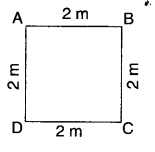(a) 8m
(b) 16m
(c) 4m
(d) none of these.

Hint:
Perimeter = 4 × 2 = 8m

Question 3.
Perimeter of a square =
(a) 4 × Length of a side
(b) 2 × Length of a side
(c) 3 × Length of a side
(d) 6 × Length of a side.

Question 4.
A page is 25 cm long and 20 cm wide. Find the perimeter of this page.
(a) 90 cm
(b) 45 cm
(c) 500 cm
(d) 5 cm.

Hint:
Perimeter = 2(25 + 20) = 90 cm

Question 5.
Perimeter of a regular hexagon =
(a) 3 × Length of a side
(b) 4 × Length of a side
(c) 5 × Length of a side
(d) 6 × Length of a side.

Question 6.
The perimeter of the figure is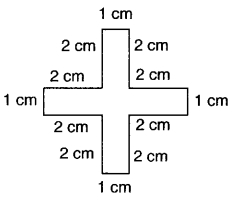(a) 5 cm
(b) 10 cm
(c) 15 cm
(d) 20 cm.

Hint:
Perimeter = 4(1 + 2 + 2) = 20cm

Question 7.
Perimeter of a rectangle =
(c) 2 × (Length + Breadth)
(d) 2 × (Length × Breadth).

Question 8.
The perimeterof the figure is(a) 20 cm
(b) 10 cm
(c) 24 cm
(d) 15 cm.

Hint:
Perimeter = 4 + 3 + 2 + 2 + 3 + 6 = 20 cm

Question 9.
Meenu wants to put a lace border all around a rectangle table cover 2 m long and 1 m wide. Find the length of the lace required by Meenu.
(a) 3 m
(b) 4 m
(c) 5 m
(d) 6m.

Hint:
Length of the lace = 2(2 + 1) = 6 m

Question 10.
Perimeter of an equilateral triangle
(a) 2 × Length of a side
(b) 3 × Length of a side
(c) 4 × Length of a side
(d) 6 x Length of a side.

Question 11.
Find the perimeter of a rectangle whose length and breadth are 9 cm and 1 cm respectively,
(a) 10 cm
(b) 20 cm
(c) 30 cm
(d) 40 cm.

Hint:
Perimeter = 2(9 + 1) = 20 cm

Question 12.
An athlete takes 10 rounds of a rectangular park, 40 m long and 30 m wide. Find the total distance covered by him.
(a) 1400 m
(b) 700 m
(c) 70 m
(d) 2800 m.

Hint:
Total distance covered = 10 × 2 × (40 + 30) = 1400 m

Question 13.
The perimeter of a regular pentagon is 10 m. Find the length of the side.
(a) 1m
(b) 2m
(c) 5m
(d) 10m

Hint:
Length of side = $$\frac { 10 }{ 5 }$$ = 2 m

Question 14.
Find the cost of fencing a rectangular park of length 10 m and breadth 5 m at the rate of? 10 per metre.
(a) ₹ 300
(b) ₹ 600
(c) ₹ 150
(d) ₹ 1200.

Hint:
Cost= 10 × 2 × (10 + 5) = ₹ 300

Question 15.
Area of a rectangle =
(c) 2 × (Length + Breadth)
(d) 2 × (Length × Breadth).

Question 16.
The perimeter of a square of side 1 m is
(a) 1 cm
(b) 2 cm
(c) 3 cm
(d) 4 m.

Hint:
Perimeter = 4 × 1 =4m

Question 17.
Area of a square =
(a) side × side
(b) 4 × Length of a side
(c) 2 × Length of a side
(d) 6 × Length of a side.

Question 18.
The perimeter of an equilateral triangle of side 1 m is
(a) 1 m
(b) 2 m
(c) 3 m
(d) 6 m.

Hint:
Perimeter = 3 × 1 = 3 m

Question 19.
The perimeter of a regular pentagon of side 1 m is
(a) 5 m
(b) 10 m
(c) 15m
(d) 20m.

Hint:
Perimeter = 5 × 1 = 5 m

Question 20.
The perimeter of an equilateral triangle is 9 m. Find the length of the side.
(a) 1 m
(b) 2 m
(c) 3 m
(d) 9 m.

Hint:
Length of side = $$\frac { 9 }{ 3 }$$ = 3 m

Question 21.
Perimeter of a regular pentagon =
(a) 4 × Length of a side
(b) 3 × Length of a side
(c) 6 × Length of a side
(d) 5 × Length of a side.

Question 22.
The perimeter of a regular hexagon of side 1 m is
(a) 3 m
(b) 2 m
(c) 4 m
(d) 6 m.

Hint:
Perimeter = 6 × 1 = 6m

Question 23.
The perimeter of the figure is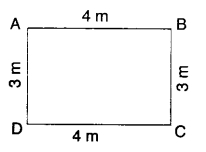(a) 12m
(b) 14m
(c) 24 m
(d) 7 m.

Hint:
Perimeter = 2(4 + 3) = 14m

Question 24.
Find the distance travelled by Sangeeta if she takes 5 rounds of a square park of side 10 m.
(a) 200 m
(b) 100 m
(c) 400m
(d) 800 m.

Hint:
Distance = 5 × 4 × 10 = 200 m

Question 25.
The perimeter of a square is 8 m. Find the length of the side.
(a) 1m
(b) 2m
(c) 4m
(d) 8m.

Length of side = $$\frac { 8 }{ 4 }$$ = 2 m